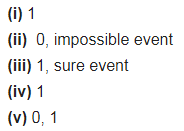# Complete the following statements:

Complete the following statements:
(i) The probability of an event E + Probability of the event ‘not E’ = ………
(ii) The probability of an event that cannot happen is ……… Such an event is called ………
(iii) The probability of an event that is certain to happen is ………. Such an event is called ………
(iv) The sum of the probabilities of all the elementary events of an experiment is …………
(v) The probability of an event is greater than or equal to …………. and less than or equal to …………